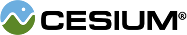#DepthFunction

#### DepthFunction() Scene/DepthFunction.js 15

Determines the function used to compare two depths for the depth test.

### Members

#### staticconstantCesium.DepthFunction.ALWAYS : Number Scene/DepthFunction.js 78

The depth test always passes.

#### staticconstantCesium.DepthFunction.EQUAL : Number Scene/DepthFunction.js 38

The depth test passes if the incoming depth is equal to the stored depth.

#### staticconstantCesium.DepthFunction.GREATER : Number Scene/DepthFunction.js 54

The depth test passes if the incoming depth is greater than the stored depth.

#### staticconstantCesium.DepthFunction.GREATER_OR_EQUAL : Number Scene/DepthFunction.js 70

The depth test passes if the incoming depth is greater than or equal to the stored depth.

#### staticconstantCesium.DepthFunction.LESS : Number Scene/DepthFunction.js 30

The depth test passes if the incoming depth is less than the stored depth.

#### staticconstantCesium.DepthFunction.LESS_OR_EQUAL : Number Scene/DepthFunction.js 46

The depth test passes if the incoming depth is less than or equal to the stored depth.

#### staticconstantCesium.DepthFunction.NEVER : Number Scene/DepthFunction.js 22

The depth test never passes.

#### staticconstantCesium.DepthFunction.NOT_EQUAL : Number Scene/DepthFunction.js 62

The depth test passes if the incoming depth is not equal to the stored depth.